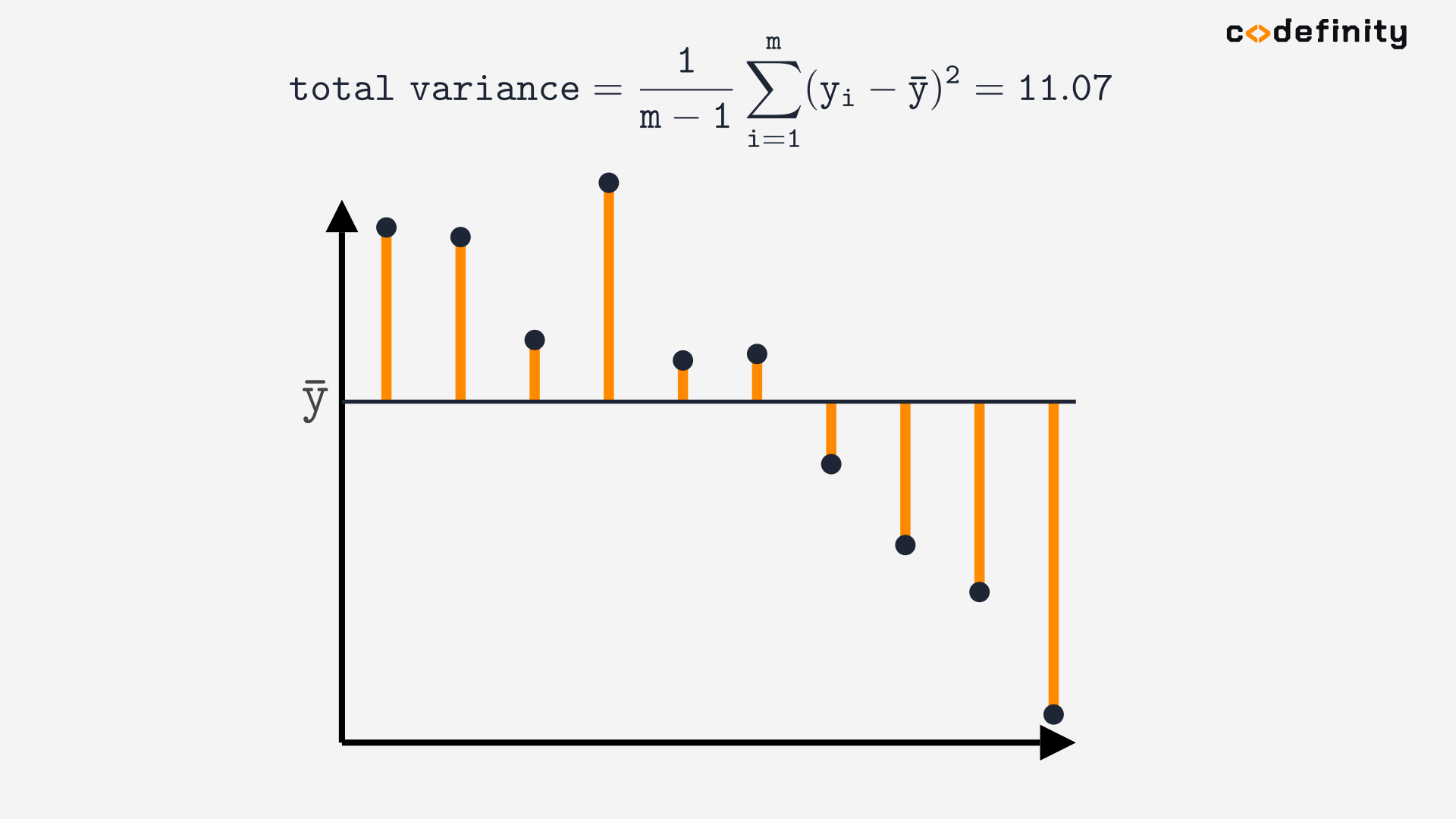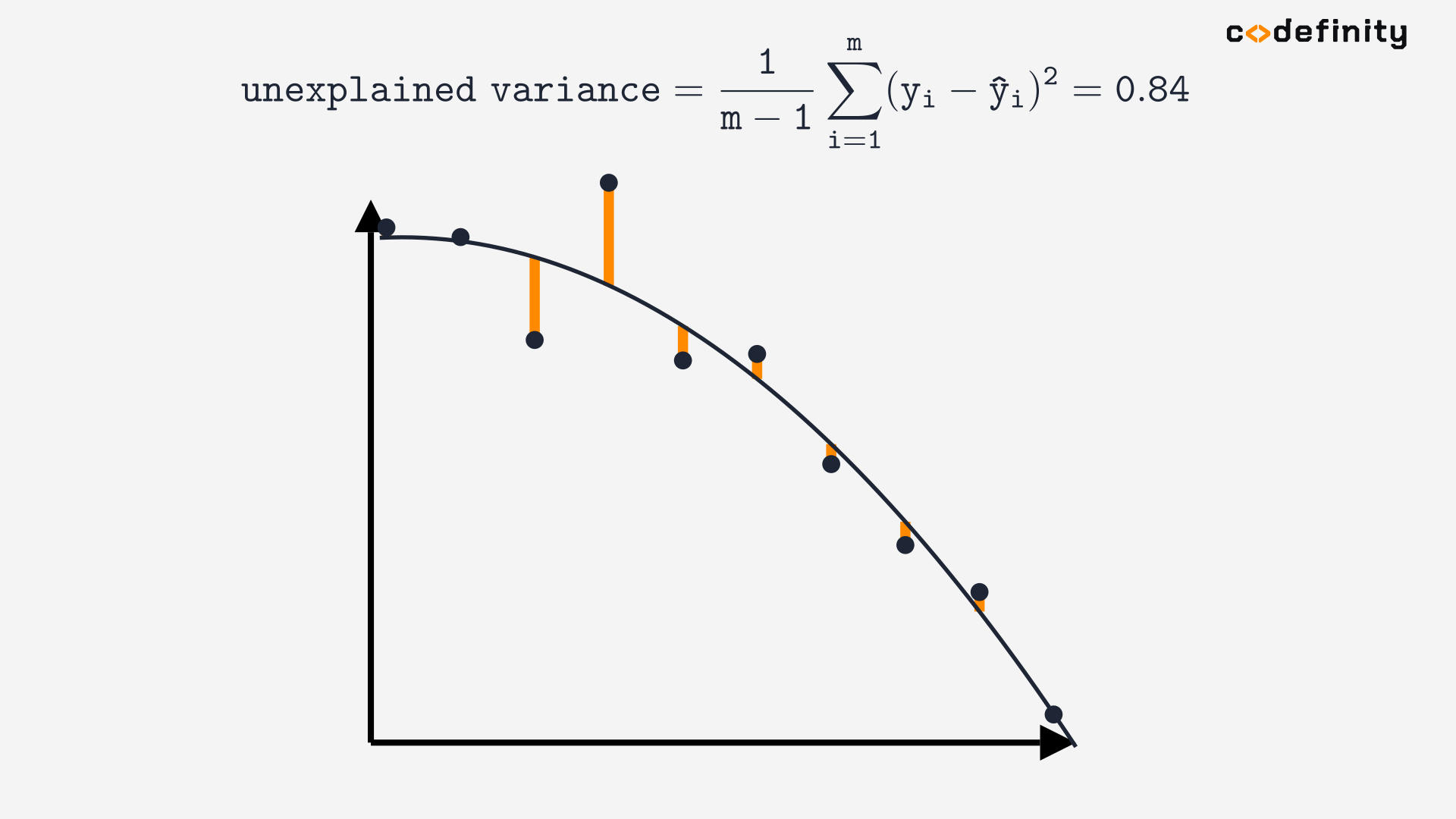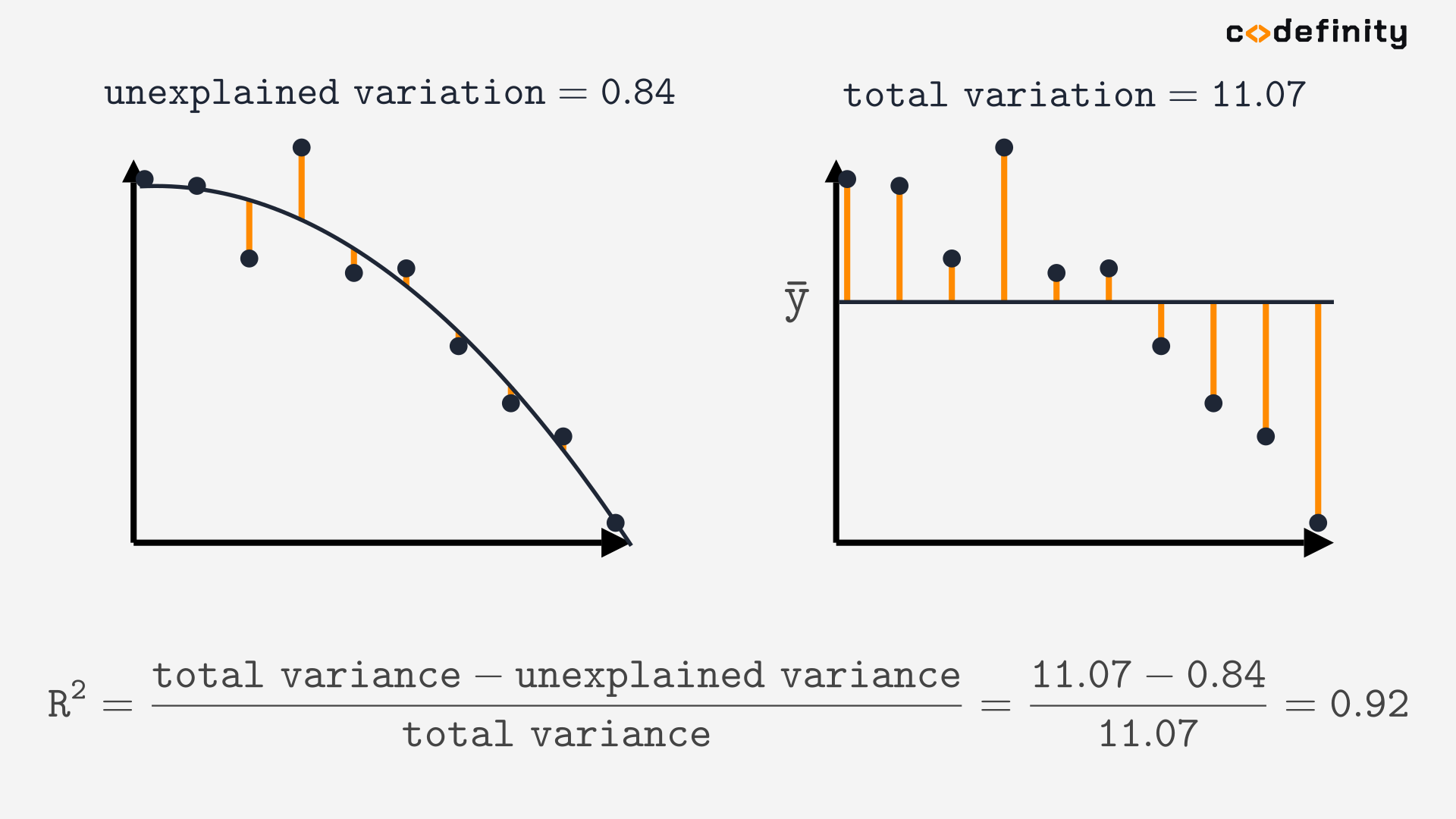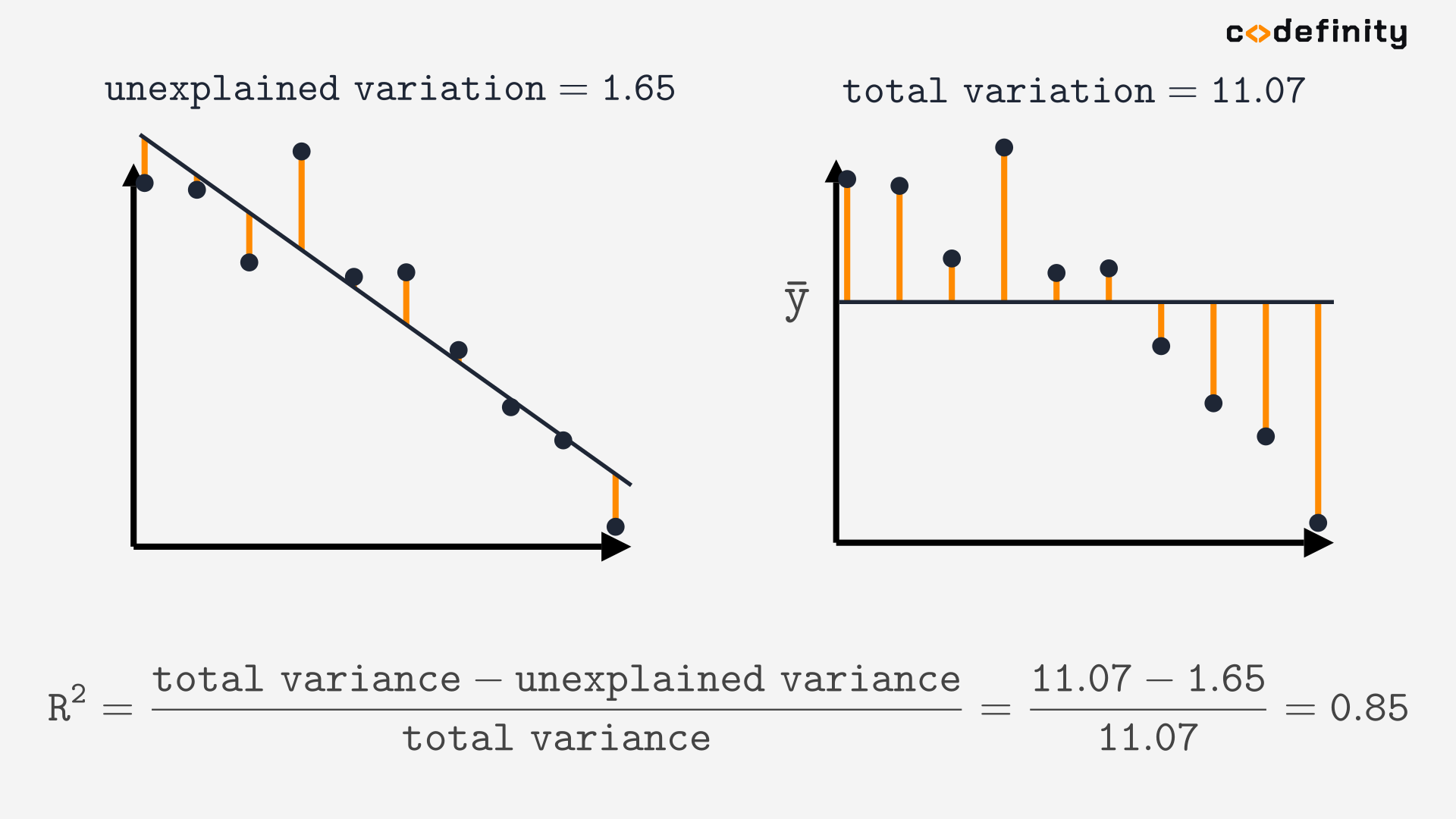Course Content

# Linear Regression with Python

Linear Regression with Python

## What is R-squared

We have covered some of the most used metrics for regression in the Metrics chapter. Those are MSE, RMSE, and MAE. They are good for comparing models, but when you build one model, you do not always understand if it is a good score for your dataset or if you need to continue trying other models.

Luckily there is a metric called R-squared that measures the model's performance on a scale from 0 to 1. R-squared calculates the proportion of the target's variance explained by the model.The problem is we cannot calculate the explained variance right away. But we can calculate unexplained variance, so let's transform the above equation to:## total variance

The total variance is just a target's variance, and we can calculate the target's variance using the sample variance formula from Statistics ( is the target's mean):Here is an example with visualization. Differences between the actual target value and the target's mean are colored orange. Just like with calculating SSR, we take the length of each orange line, square it, and add it to the sum, but now we also divide the result by m-1. Here we got a total variance of 11.07.## unexplained variance

Now we need to calculate the variance that is unexplained by the model. If the model explained the whole variance, all the points would lie on the built regression line. That is seldom the case, so we want to calculate the target's variance but now with respect to the regression line instead of the mean. We will use the same formula but replace with the model's predictions.Here is an example with visualization:Now we know everything to calculate the R-squared:We got an R-squared score of 0.92 which is close to 1, so we have a great model. Let's also calculate R-squared for one more model.The R-squared is lower since the model underfits the data a little bit.

## R-squared in Python

The `sm.OLS` class calculates the R-square for us. We can find it in the `summary()` table here.To sum it up, R-squared is a metric for a regression. It can take values from 0 to 1. Unlike other metrics like MSE/MAE, a higher value is better(unless the model overfits). You can find the R-squared in the `summary()` table of the `sm.OLS` class.

Everything was clear?

Section 4. Chapter 3